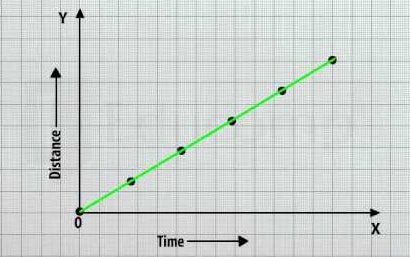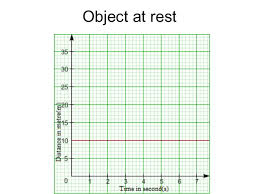Wednesday , October 5 2022# NCERT 7th Class (CBSE) Science: Time and Motion

#### Question: Graphic represent the nature of the distance-time graph of motion of the given object –

Answer: (1) An athlete running at a constant speed.(2) A train waiting at a station for another train pass by.#### Question: Differentiate between periodic and non periodic motion.

Answer: Differentiate between periodic and non periodic motion are:

Periodic motion
Non periodic motion
1. In periodic motion the object always comes after a time period.
1. In non periodic motion object does not always comes after a time period.
2. For Eg-pendulum of a clock, Revolution and Rotation of the earth.
2. For Eg- Time taken to go to school.

#### Question: Define speed Mention SI unit of speed.

Answer: The distance covered by a body in unit time is called speed. Usually speed is denoted as km/h, but the SI unit of speed is metre per second (m/s). The unit of speed can be converted in km/h, m/h.

#### Question: The length of the simple pendulum is increased? What will happen to time period?

Answer: If the length of the simple pendulum is increased the oscillations will a slow down.

#### Question: The mass of the bob is doubled? What will happen to timeperiod?

Answer: The time will remain unchanged.

## Sources of Energy: 10th Science Chapter 14

Class: 10th Class Subject: Science Chapter: Chapter 14: Sources of Energy Quiz: – Questions MCQs: …